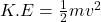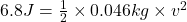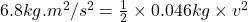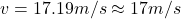## A toy cannon launches a 46-g golf ball straight up into the air with a kinetic energy of 6.8 J. What must the ball’s velocity be

Question

A toy cannon launches a 46-g golf ball straight up into the air with a kinetic energy of 6.8 J. What must the

ball’s velocity be as it leaves the cannon?

A. 0.54 m/s

B. 0.79 m/s

C. 17 m/s

D. 80 m/s

in progress 0
2 months 2021-07-25T17:08:13+00:00 1 Answers 3 views 0

1. Answer : The correct option is, (C) 17 m/s

Explanation :

Formula used :where,

K.E = kinetic energy = 6.8 J

m = mass of object = 46 g = 0.046 kg    (1 kg = 1000 g)

v = velocity

Now put all the given values in the above formula, we get:Therefore, the ball’s velocity be as it leaves the cannon is, 17 m/s